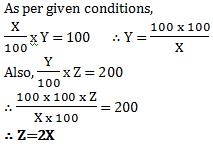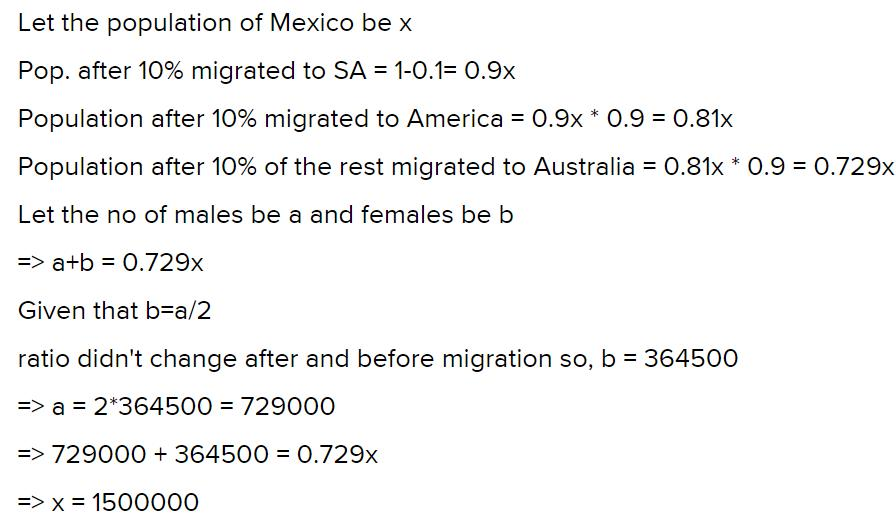Courses

Practice Test: Percentages- 1

10 Questions MCQ Test IBPS Clerk Prelims - Study Material, Mock Tests | Practice Test: Percentages- 1

Description
Attempt Practice Test: Percentages- 1 | 10 questions in 20 minutes | Mock test for Banking Exams preparation | Free important questions MCQ to study IBPS Clerk Prelims - Study Material, Mock Tests for Banking Exams Exam | Download free PDF with solutions
QUESTION: 1

What is 10% of 20% of 25% of 100?

Solution:

25% of  100 = (25/100) x 100 = 25
20% of 25% of 100 = 20% of 25 = (20/100) x 25 = 5
10% of 20% of 25% of 100 = 10% of 5 = (10/100) x 5 = 0.50

QUESTION: 2

30% of a% of b is 25% of b% of c. Which of the following is c?

Solution:

Given:
a% of b = (a/100) x b = ab/100
30% of a% of b = 30% of ab/100
= (30/100) x ab/100
= 3ab/1000

Also Given:
b% of c = (b/100) x c = bc/100
25% of b% of c = 25% of bc/100
= (25/100) x bc/100
= bc/400

Now ATQ:
(3ab/1000) = (bc/400)

⇒ 3a/10 = c/4
⇒ c = 12a/10
⇒ c = 1.20a

QUESTION: 3

If X% of Y is 100 and Y% of Z is 200, find a relation between X and Z.

Solution:QUESTION: 4

In an election between 2 candidates, Walter gets 80% of the total valid votes. If the total votes were 12000, what is the number of valid votes that the other candidate Jesse Pinkman gets if 15% of the total votes were declared invalid?

Solution:

Total Valid votes = 85% of 12000 = 10200.
Jesse Pinkman would get 10200 – 8160 = 2040 Votes.

QUESTION: 5

A number is mistakenly divided by 2 instead of being multiplied by 2. Find the percentage change in the result due to this mistake.

Solution:

Let the number be 100. Then, 200 should be the correct outcome. But instead, the value got is 50.
Change in value = 200 – 50 = 150.
The percentage change in the value = 150 X 100 / 200 = 75%.
Alternatively, you could think of this as the number being ‘x’ and the required result being 2x and the derived result being 0.5x.

Hence, the percentage change in the result is 1.5x X 100 / 2x. Clearly, the value would be 75%. (Note: In this case, the percentage change in the answer does not depend on the value of ‘x’).

QUESTION: 6

In a mixture of 100 litres of milk and water, 25% of the mixture is milk. How much water should be added to the mixture so that milk becomes 20% of the mixture?

Solution:

From the first statement we get that out of 100 litres of the mixture, 25 litres must be milk.
Since we are adding water to this and keeping the milk constant, it is quite evident that 25 litres of milk should correspond to 20% of the total mixture.
Thus, the amount in the total mixture must be 125, which means we need to add 25 litres of water to make 100 litres of the mixture.

QUESTION: 7

10% of Mexico’s population migrated to South Asia, 10% of the remaining migrated to America and 10% of the rest migrated to Australia. If the female population, which was left in Mexico, remained only 3,64,500, find the population of Mexico City before the migration and its effects if it is given that before the migration the female population was half the male population and this ratio did not change after the migration?

Solution:QUESTION: 8

If the length, breadth and height of a cube are decreased, decreased and increased by 5%, 5% and 20%, respectively, then what will be the impact on the surface area of the cube (in percentage terms)?

Solution:

Assume the initial surface area as 100 on each side. A total of 6 such surfaces would give a total surface area of 600.

Two surface areas would be impacted by the combined effect of length and breadth, two would be affected by length and height and two would be affected by breadth and height.

Thus, the respective surface areas would be (90.25 twice, 114 twice and 114 twice) Thus, new surface area = 180.5 + 456 = 636.5
A percentage increase of 6.0833%

QUESTION: 9

The price of raw materials has gone up by 15%, labour cost has also increased from 25% of the cost of raw material to 30% of the cost of raw material. By how much percentage should there be a reduction in the usage of raw materials so as to keep the cost the same?

Solution:

Let the initial price of raw materials be 100.
The new cost of the same raw material would be 115.
The initial cost of labour would be 25 and the new cost would be 30% of 115 = 34.5

The total cost initially would be 125.
The total cost for the same usage of raw material would now be: 115 + 34.5 = 149.5

This cost has to be reduced to 125. The percentage reduction will be given by 24.5 / 149.5 = 17 % approx.

QUESTION: 10

There are three galleries in a coal mine. On the first day, two galleries are operative and after some time, the third gallery is made operative. With this, the output of the mine became half as large again. What is the capacity of the second gallery as a percentage of the first, if it is given that a four-month output of the first and the third galleries was the same as the annual output of the second gallery?

Solution:

The third gallery making the capacity ‘half as large again’ means an increase of 50%.

Further, it is given that: 4(first + third) = 12 (second) In order to get to the correct answer, try to fit in the options into this situation.

(Note here that the question is asking you to find the capacity of the second gallery as a percentage of the first.)

If we assume option (a) as correct – 70% the following solution follows:
If the second is 70, then first is 100 and the first + second is 170. Then third will be 85 (50% of first + second).

Then the equation:
4 X (100 + 85) should be equal to 12 X 70
But this is not true.

Through trial and error, you can see that the third option fits correctly.
4 X (100 + 80) = 12 X 60.
Hence, it is the correct answer.Use Code STAYHOME200 and get INR 200 additional OFF Use Coupon Code

Track your progress, build streaks, highlight & save important lessons and more!

Similar ContentRelated tests